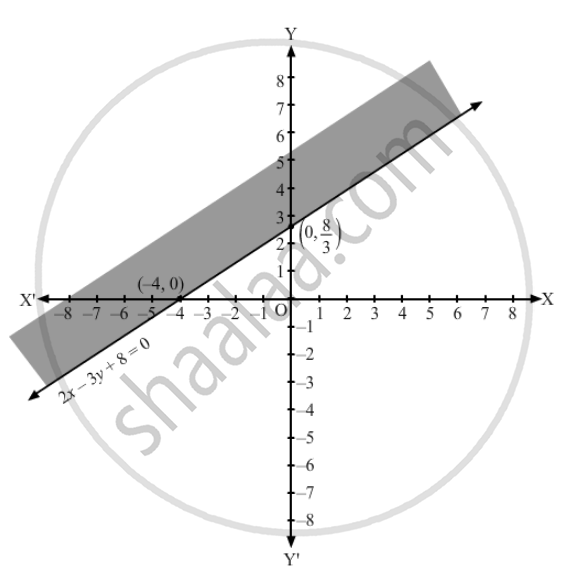Advertisement Remove all ads

# Represent to Solution Set of Each of the Following Inequations Graphically in Two Dimensional Plane: 3x − 2y ≤ X + Y − 8 - Mathematics

Represent to solution set of each of the following inequations graphically in two dimensional plane:

3x − 2y ≤ x + y − 8

Advertisement Remove all ads

#### Solution

Converting the inequation to equation, we obtain 3x$-$ 2y$-$ x$-$y + 8 = 0, i.e 2x$-$ 3y+ 8= 0
Putting  y =0 and x = 0 in this equation, we obtain x = $-$and y = 8/3 respectively.
So, this line meets the x-axis at ($-$4, 0) and y-axis at (0,8/3).
We plot these points and join them by a thick line.
This divides the xy plane into two parts.
To determine the region represented by the given inequality, consider point O(0,0).
Clearly, (0,0) does not satisfy the inequality.
So, the region that does not contain the origin is represented by the given inequality.
Hence, the shaded region is the solution to the inequation.Is there an error in this question or solution?
Advertisement Remove all ads

#### APPEARS IN

RD Sharma Class 11 Mathematics Textbook
Chapter 15 Linear Inequations
Exercise 15.5 | Q 10 | Page 28
Advertisement Remove all ads
Advertisement Remove all ads
Share
Notifications

View all notifications

Forgot password?